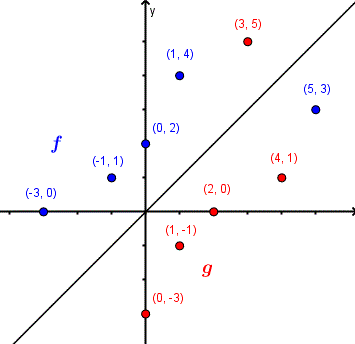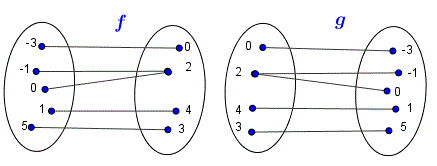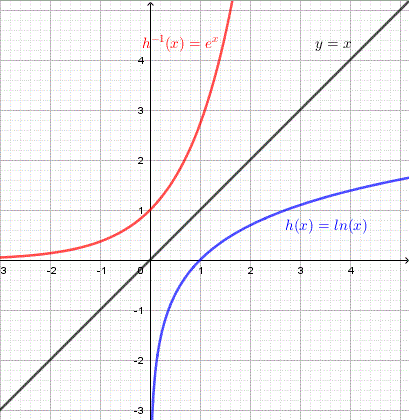# Inverse Function

First, the definition and properties of inverse function are reviewed. Then the graphs of of one to one functions functions and their inverses are invetsigated graphically.
More tutorials on finding inverse functions are included.

## Definition, properties of inverse functions

### 1 - Definition

Let function f be defined as a set of ordered pairs as follows:
f = { (-3 , 0) , (-1 , 1) , (0 , 2) , (1 , 4) , (5 , 3)}
The inverse of function f is defined by interchanging the components (a , b) of the ordered pairs defining function f into ordered pairs of the form (b , a). Let g be the inverse of function f; g is then given by
g = {(0 , - 3) , (1 , - 1) , (2 , 0) , (4 , 1) , (3 , 5)}Figure 1. The definition of the inverse of a function using Venn diagrams The plots of the set of ordered pairs of function f and its inverse g are shown below.Figure 2. The definition of the inverse of a function using graphs Function f and its inverse g are reflection of each other on the line y = x.

### 2 - Inverse Function Notation

The inverse function, denoted f -1, of a one-to-one function f is defined as

f -1(x) = {(y,x) | such that y = f(x)}

Note: The -1 in f
-1 must not be confused with a power.

If function f is not a one-to-one then it does not have an inverse. More discussions on one to one functions will follow later.

### 3 - Domain and Range of a Function and its Inverse

Let function f be defined by the set of ordered pairs as follows:
f = {(1,0),(4,5),(6,9)}
According the definition above, the inverse function of f is given by:
f -1 = {(0,1),(5,4),(9,6)}
The domain of f is equal to the range of f -1 and the range of f is equal to the domain of f -1.

### 4 - Composition of a Function and its Inverse

• f(f -1(0)) = f(1) = 0
• f(f -1(5)) = f(4) = 5
• f(f -1(9)) = f(6) = 9
and
• f -1(f(1)) = f -1(0) = 1
• f -1(f(4)) = f -1(5) = 4
• f -1(f(6)) = f -1(9) = 6

and in general
f(f
-1(x)) = x , x in the domain of f -1

f
-1(f(x)) = x , x in the domain of f

### 5 - What is the inverse function needed for?

In some situations we now the output of a function and we need to find the input and that is where the inverse function is used.
Example: Find x such that 0 < x < π/2 and
sin(x) = 0.2
x = arcsin(0.2) , here arcsin is the inverse of sin(x).

### 6 - Which functions have an inverse function (invertible functions) ?

Let function f be defined as a set of ordered pairs as follows:
f = { (-3 , 0) , (-1 , 2) , (0 , 2) , (1 , 4) , (5 , 3)}
The inverse of function f is obtained by interchanging the components (a , b) of the ordered pairs defining function f into ordered pairs of the form (b , a). Let g be the inverse of function f; g is then given by
g = {(0 , - 3) , (2 , - 1) , (2 , 0) , (4 , 1) , (3 , 5)}
Below are shown the Venn diagrams of function f and its inverse g and we note that g is
not a function (input 2 has two outputs -1 and 0). We say that function f is not invertible (does not have an inverse) because it is not a one-to-one functions or only one to one functions have an inverse.Figure 4. The inverse of function f is not a function Below are shown the graphs of f and its inverse g and we note again that g(2) = 0 and g(2) = - 1 and a vertical line would pass by both points (2 , 0) and (2 , -1) and therefore g is not a function.Figure 5. The inverse of function f is not a function using graphs More on one-to-one functions.

### 7 - Important properties of a function and its inverse

1) The domain of f -1 is the range of f
2) The range of f
-1 is the domain of f
3) (f
-1o f)(x) = x for x in the domain of f
4) (f
o f -1)(x) = x for x in the domain of f -1
5) The graphs of f and f
-1 are reflection of each other on the line y = x
More on the
properties of inverse functions is included in this website.

### 8 - Graphical investigation of the properties of the inverse functions

The graphs of three functions (in blue) and their inverses (in red) are shown below. examine the graphs and answer the questions below.### 9 - Questions

1) For each function, select some points on the graph of f and determine their coordinates, then find their inverses on the graph of the inverse function.
2) Why are the graphs of a function and its corresponding inverse a reflection of each other on the line y = x?
3) For each function, find its domain and range and deduce the domain and range of the corresponding inverse then verify your results graphically.

## Answers to the Above Questions

1) If (a,b) is a point on the graph of f then point (b,a) is a point on the graph of f-1
a) F(x) = x
2 , x ≥ 0.
points on the graph of f: (0,0) , (1,1) , (2,4)
corresponding points on f
-1(x) : (0,0), (1,1) and (4,2)

b) g(x) = x
3.
points on the graph of g: (-1,-1) , (0 , 0) , (1,1) , (2,8)
corresponding points on g
-1(x) : (-1,-1) , (0,0), (1,1) and (8,2)

c) h(x) = ln(x).
points on the graph of h: (1,0) , (4 , 1.4)
corresponding points on h
-1(x) : (0,1) , (1.4,4)

2) From the definition of the inverse function given above, if point (a,b) is on the graph of function f then point (b,a) is a point on the graph of f
-1. But points (a,b) and (b,a) are reflection of each other on the line y = x and therefore the whole graph of a function and its inverse are reflection of each other on the line y = x.

3) a) f(x) = x
2 , x ≥ 0
The domain of f is given by the interval: [0 , + ∞)
The range of f is given by the interval: [0 , + ∞)
One of the properties of a function and its inverse is that the domain of f is the range of f
-1 and the range of f is the domain of f-1. Hence the domain of f-1 is given by the interval [0 , + ∞)
and the range of f
-1 is given by the interval [0 , + ∞).

b) g(x) = x
3
The domain of g is given by the interval: (- ∞ , + ∞)
The range of g is given by the interval: (- ∞ , + ∞)
Hence The domain of g
-1 is given by the interval: (- ∞ , + ∞)
The range of g
-1 is given by the interval: (- ∞ , + ∞)

c) h(x) = ln(x)
The domain of h is given by the interval: (0 , + ∞)
The range of h is given by the interval: (- ∞ , + ∞)
Hence
The domain of h
-1 is given by the interval: (- ∞ , + ∞)
The range of h
-1 is given by the interval: (0 , + ∞)

### 10 - Exercises

Exercise 1:
a) Find the domain and range of function f defined by
f = {(-4,2),(-3,1),(0,5),(2,6)}
b) Find the inverse function of f and its domain and range.
Exercise 2:
Which of these functions do not have an inverse?
f = {(-1,2),(-3,1),(0,2),(5,6)}
g = {(-3,0),(-1,1),(0,5),(2,6)}
h = {(2,2),(3,1),(6,5),(7,1)}

Exercise 1:
a) domain of f = {-4,-3,0,2} and range of f = {2,1,5,6}
b) inverse of f = {(2,-4),(1,-3),(5,0),(6,2)}
domain of inverse of f = {2,1,5,6} and range of inverse of f = {-4,-3,0,2}.
Exercise 2:
Functions f and h are not one to one functions and therefore do not have inverses.

### More References and links to the Inverse Functions

Find inverse of exponential functions
Inverse Functions Graphing
Applications and Use of the Inverse Functions
Find the inverse of a Function - Questions
Find the Inverse Function (1) - Tutorial.
Find the Inverse Function (2) - Tutorial
Definition of the Inverse Function - Interactive Tutorial
Find Inverse Of Cube Root Functions.
Find Inverse Of Square Root Functions.
Find Inverse Of Logarithmic Functions.
Find Inverse Of Exponential Functions. Properties of Inverse Functions